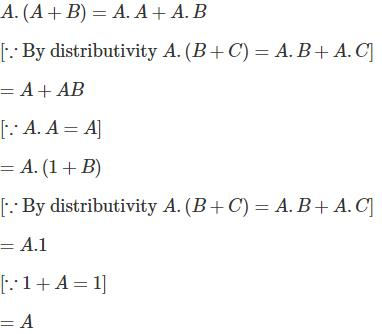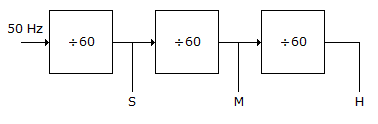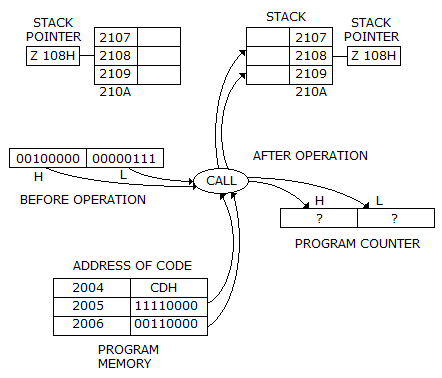Courses

# Test: Digital Electronics - 7

## 25 Questions MCQ Test Digital Electronics | Test: Digital Electronics - 7

Description
This mock test of Test: Digital Electronics - 7 for Electrical Engineering (EE) helps you for every Electrical Engineering (EE) entrance exam. This contains 25 Multiple Choice Questions for Electrical Engineering (EE) Test: Digital Electronics - 7 (mcq) to study with solutions a complete question bank. The solved questions answers in this Test: Digital Electronics - 7 quiz give you a good mix of easy questions and tough questions. Electrical Engineering (EE) students definitely take this Test: Digital Electronics - 7 exercise for a better result in the exam. You can find other Test: Digital Electronics - 7 extra questions, long questions & short questions for Electrical Engineering (EE) on EduRev as well by searching above.
QUESTION: 1

Solution:
QUESTION: 2

Solution:
QUESTION: 3

### The hexadecimal number 64 AC is equivalent to decimal number

Solution:
QUESTION: 4

A binary with a digits, all of which are unity has the value

Solution:
QUESTION: 5

In a NAND SR latch S = R = 1. Then

Solution:
QUESTION: 6

A microprocessor with a 16-bit address bus is used in a linear memory selection configuration (i.e., Address bus lines are directly used as chip selects of memory chips) with 4 memory chips. The maximum addressable memory space is

Solution:
QUESTION: 7

A (A + B) =

Solution:QUESTION: 8

The logic represented by the abbreviation ECL is

Solution:
QUESTION: 9

One of the function of the Sio2 layer in an IC is to

Solution:
QUESTION: 10

Unlimited number of ICs 7490, 78492 and 7493 are available. Which of these could be used to build the dividse-by-60 circuit of figureSolution:
QUESTION: 11

In a shift register the data is loaded in one operation but shifted out one bit at a time, The shift register is

Solution:
QUESTION: 12

In CCD

Solution:
QUESTION: 13

The contents of the program counter after the call operation point to the first instruction on theSolution:
QUESTION: 14

Dual slope ADC uses an op amp comparator.

Solution:
QUESTION: 15

The output of the 74 series of TTL gates is taken from a BST in

Solution:
QUESTION: 16

Assertion (A): Demorgan's first theorem replaces a NOR gate by bubbled AND gate.

Reason (R): Double complement of a variable equals the variable.

Solution:
QUESTION: 17

A flip-flop circuit is

Solution:
QUESTION: 18

Assertion (A): The use of 2's complement has simplified the computer hardware for arithmetic operations

Reason (R): 2's complement is obtained by adding 1 to 1's complement.

Solution:
QUESTION: 19

How many lines are there in address bus of 8085 μp?

Solution:
QUESTION: 20

Consider the following statements Timer 555 can be used as

1. monostable multivibrator
2. bistable multivibrator
3. astable multivibrator
Which of the above statements are correct?
Solution:
QUESTION: 21

Solution:
QUESTION: 22

How many AND gates are required to realize Y = CD + EF + G?

Solution:

To realize Y = CD + EF + G, two AND gates are required and two OR gates are required.

QUESTION: 23

In floating point representation, the accuracy is

Solution:
QUESTION: 24

The PC contains 0450 H and SP contains 08D 6 H. What will be content of P and SP following a CALL to subroutine at location 02 AFH?

Solution:
QUESTION: 25

An n bit ADC using Vr as reference has a resolution (in volts) of

Solution: